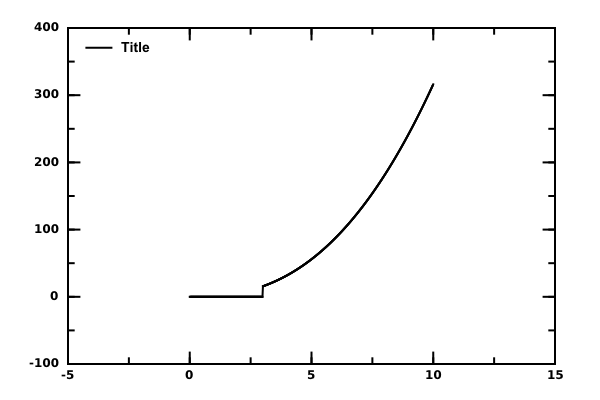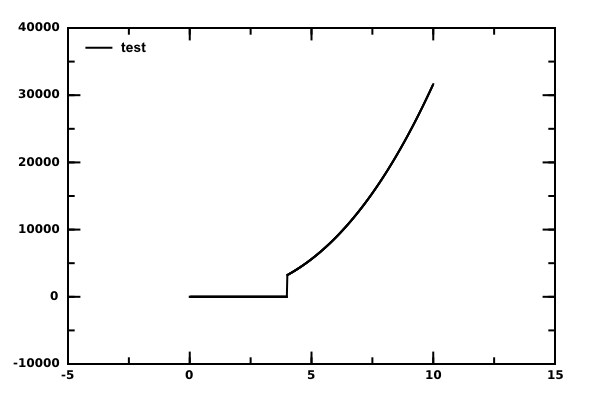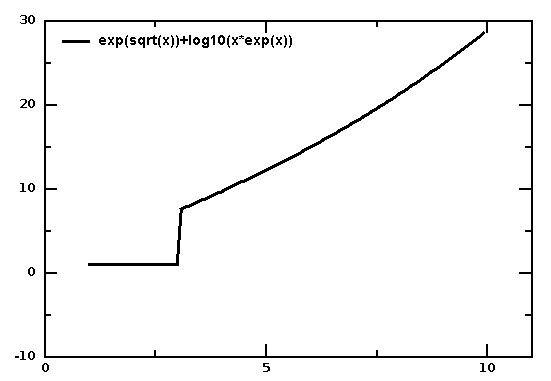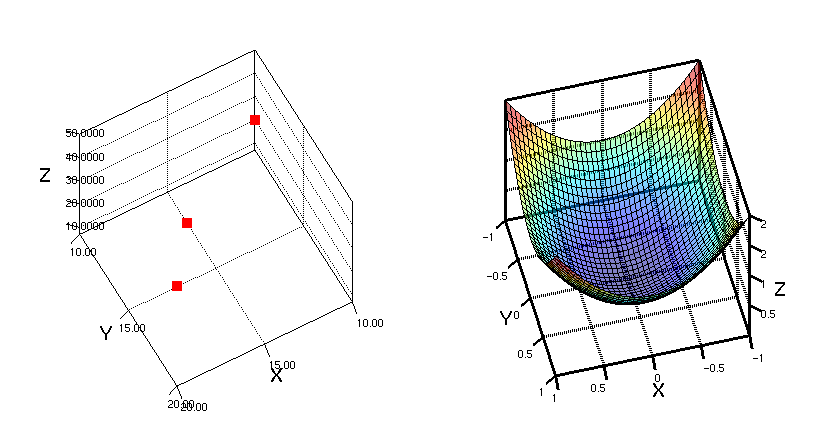# DMelt:Math/3 Non Parametric Functions

Limitted access. First login to DataMelt if you are a full DataMelt member. Then login to HandWiki as a user.

# Non-parametric functions

The most flexible way to draw functions is to use codding with objects and calling third-party Java libraries directly, instead of defining a function using strings as it was done forjhplot.F1D orjhplot.F2D classes. Here we will show how to create functions completely programmically using any arbitrary definition. Below we will show several approaches.

## Extending FNon Java class

Let us consider a function of the form:

if x>3: y=x*x*sqrt(x)
if x<3: y=0

To construct the mathematical functions defined above and plot it, one can build a new class by extending the classjhplot.FNon. You will need to overwrite the method "value()" that returns the calculated value.

This example shows how to do this assuming no free parameters:

Here we used functions from the Java classjava.lang.Math with mathematical functions. Note that x indicates a variable (0 means that the dimension of this function is one). As you can see, we define the function using Python codding, and then use it as input for the classjhplot.F1D that can be plotted. The result of this script is shown below.You can extend this example by adding two free parameters, p and p. This example shows how to do this:

with the image isNote that you can also construct functions in a similar way using Java codding.

## Using user-defined steps

Here is an example of some complex function of the form:

if x>3: exp(sqrt(x))+log10(x*exp(x))
if x<3: 1

This time we will draw this function using Java methods from the classjava.lang.Math:

from jhplot import *
from java.lang.Math import *

def func(min,max):
f=P1D("exp(sqrt(x))+log10(x*exp(x))")
step=(max-min)/100.0 # define step
for i in range(100):
x=min+step*i
y=exp(sqrt(x))+log10(x*exp(x))  # get value of function
if (x<3): y=1
return f

c1 = HPlot()   # plot
c1.visible(); c1.setAutoRange()
p=func(1,10)   # plot between 1 and 100
p.setStyle('l')
p.setPenWidth(3)
c1.draw(p)

As you see, it takes more codding to create such function, but the function can be as complex as you want. In this example we used the classjhplot.P1D which keeps X-Y values and used 100 steps for evaluation. Note that you can call various special functions in this example if you will import appropriate Java class.

The result of this script is:## Using Java coding

Since all classes are implemented in Java, one can use the standard Java syntax to draw functions:

## Using Python definition

So far we have considered functions that can be contracted using Jython, as well as Java, and any other Java scripting language. The reason is that we used only Java classes. Next we will consider an approach that can be used using Python-only approach.

Let us illustrate this approach here. We will plot the function:

$\displaystyle{ if x\gt 0 \quad y=a*x^{2}+b }$

$\displaystyle{ x=0 \quad y=0 }$

$\displaystyle{ if x\lt 0 \quad y=a*x*cos(x)*b }$

where a and b are constants. To build such function using a single string is not easy, but it is possible to construct such function using the standard Jython. Below we will construct such function and make a plot for a=20 and b=30:

The output is:In a similar way one can build any function with several variables. Note that we create a class of this function "cmt", where v denotes variable "x". For several variables, say x and y, one should use v (for x) and v for y. Analogously, one can use the same approach for x variable.

A second approach to show functions is discussed in the section Distribution Function where we fill function using a third-party class. You can find more example how to create mathematical functions in 3D programmically in later sections.